Fast simulation algorithm for area target visibility using remote sensing satellites
E Zhibo, LI JunfengSchool of Aerospace Engineering, Tsinghua University, Beijing 100084
Abstract: Fast calculations of area target visibility are important for satellite mission scheduling. This paper presents a fast simulation algorithm to compute the visibility of area targets by remote sensing satellites. A method was developed to judge the visibility of a ground point from a satellite based on the satellite's view field and the geometric relation between the satellite and the ground point. Then, the in-view period of a single point was obtained using large time step discretization and a binary search. The in-view period of all the discrete boundary points was then used to determine the visible time window for an area target. Numerical results show that the algorithm is efficient and accurate with the error between the algorithm and satellite tool kit (STK) predictions of aboutt 0.01 s.
Key words: remote sensing satellites     area target     visibility     fast simulation algorithm

1 卫星与地面点几何分析图 1 卫星的可视范围

1.1 卫星与地面点的位置关系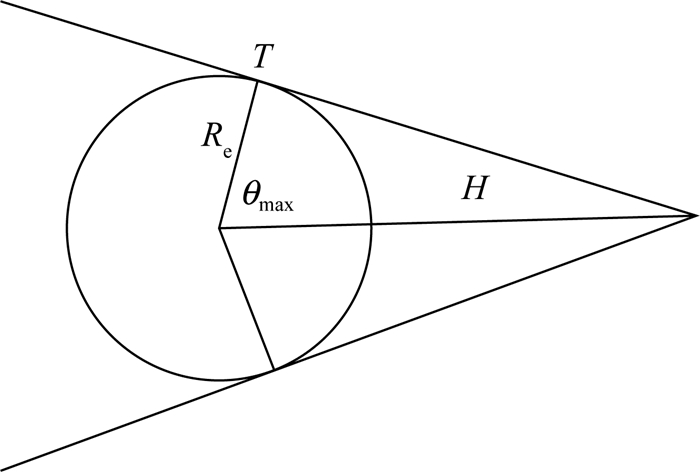图 2 卫星与地球位置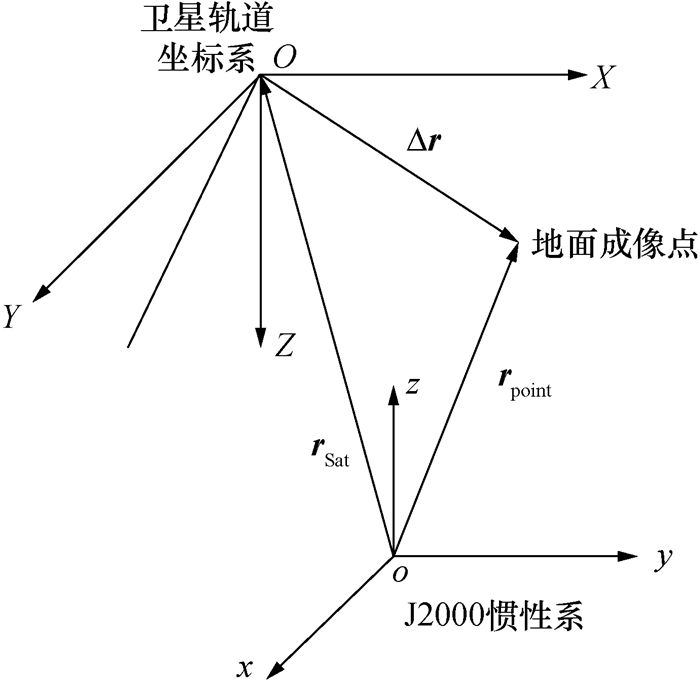图 3 卫星与地面点相对位置关系

 $\Delta \mathit{\pmb{r}} = {\mathit{\pmb{r}}_{{\rm{point}}}} - {\mathit{\pmb{r}}_{{\rm{Sat}}}}.$ (1)

J2000惯性系到卫星轨道坐标系的转换矩阵可表示为

 $\begin{array}{l} \;\;\;\;\;\;\;\;\;{\mathit{\pmb{L}}_{oi}} = {\mathit{\pmb{L}}_y}\left( { - \left( {\frac{\pi }{2} + u} \right)} \right){\mathit{\pmb{L}}_x}\left( {\left( { - \left( {\frac{\pi }{2} - i} \right)} \right){\mathit{\pmb{L}}_z}\left( \Omega \right)} \right) = \\ \left[ \begin{array}{l} - {\rm{cos\Omega sin}}{u} - {\rm{sin\Omega cos}}{i}{\rm{cos}}{u}\;\;\;\;{\rm{cos\Omega cos}}{i}{\rm{cos}}{u} - {\rm{sin\Omega sin}}{u}\;\;\;\;\;\;\;\;{\rm{cos}}{u}{\rm{sin}}{i}\\ \;\;\;\;\;\;\;\;\;\;\; - {\rm{sin\Omega sin}}{i}\;\;\;\;\;\;\;\;\;\;\;\;\;\;\;\;\;\;\;\;\;\;\;\;\;\;\;\;\;{\rm{cos\Omega sin}}{i}\;\;\;\;\;\;\;\;\;\;\;\;\;\;\;\;\;\;\;\;{-\rm{cos}}{i}{\rm{}}\\ \;\;{\rm{sin\Omega cos}}{i}{\rm{sin}}{u}{-\rm{cos\Omega cos}}{u}\;\;\;\;\;{-\rm{sin\Omega cos}}{u}{-\rm{cos\Omega cos}}{i}{\rm{sin}}{u}\;\;\;\;\;\;\;{-\rm{sin}}{i}{\rm{sin}}{u} \end{array} \right]{\rm{.}} \end{array}$ (2)

 $\Delta {\mathit{\pmb{r}}_{{\rm{orbit}}}} = {\mathit{\pmb{L}}_{oi}}\cdot\Delta {\mathit{\pmb{r}}}.$ (3)

1.2 圆锥视场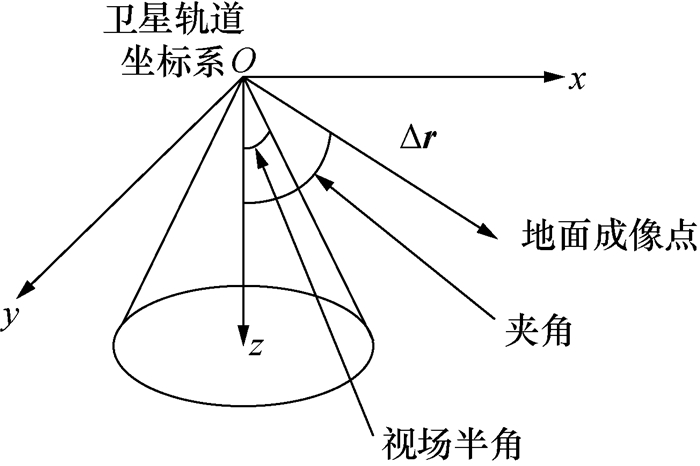图 4 圆锥视场

 $\theta = {\rm{arccos}}\frac{{\Delta {\mathit{\pmb{r}}}\cdot{\mathit{\pmb{e}}_z}}}{{\left\| {\Delta {\mathit{\pmb{r}}}} \right\|}}.$ (4)

1.3 矩形视场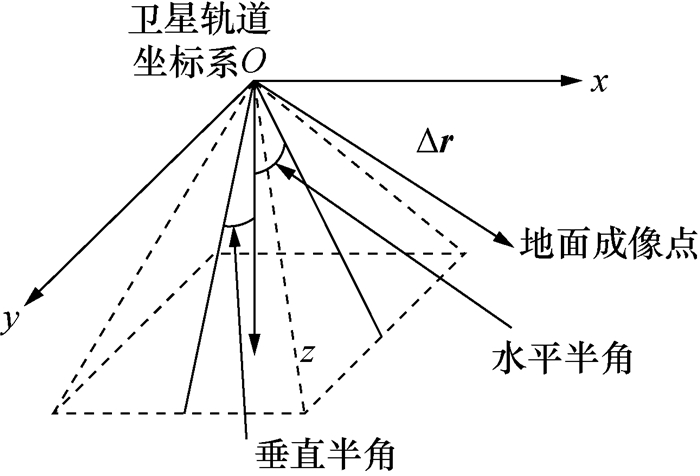图 5 矩形视场

 ${\theta _{xz}} = {\rm{arccos}}\frac{{\Delta {\mathit{\pmb{r}}_{xz}}\cdot{\mathit{\pmb{e}}_z}}}{{\left\| {\Delta {\mathit{\pmb{r}}_{xz}}} \right\|}} < {\theta _v},$ (5)
 ${\rm{ }}{\theta _{yz}} = {\rm{arccos}}\frac{{\Delta {\mathit{\pmb{r}}_{yz}}\cdot{\mathit{\pmb{e}}_z}}}{{\left\| {\Delta {\mathit{\pmb{r}}_{yz}}} \right\|}} < {\theta _h}.$ (6)
2 卫星对地面点的可见性计算

2.1 粗略计算

2.2 精确计算

3 卫星对区域目标的可见性计算

 $t{S_{{\rm{AB}}}} = {\rm{min}}\left\{ {t{S_{{N_i}}}} \right\}，$ (7)
 $t{E_{{\rm{AB}}}} = {\rm{min}}\left\{ {t{E_{{N_i}}}} \right\}.$ (8)

 $t{S_{{\rm{Area}}}} = {\rm{min}}\left\{ {t{S_{{E_i}}}} \right\}，$ (9)
 $t{E_{{\rm{Area}}}} = {\rm{max}}\left\{ {t{E_{{E_i}}}} \right\}.$ (10)

4 数值模拟

 参数 参数值 半长轴/km 7 128.14 离心率 0.0 倾角/(°) 19.925 升交点赤经/(°) 0.0 近地点幅角/(°) 219.484 平近点角/(°) 326.698

 顶点 经度/(°) 纬度/(°) 1 110.0 22.0 2 110.0 12.0 3 118.0 12.0 4 118.0 22.0

 视场类型 快速算法/s 传播算法/s 圆锥 1.093 214.983 矩形 1.406 289.893

 视场类型 编号 可见窗口开始时刻 持续时间/s 圆锥 1 2020-12-18 03:16:24.410 260.006 2 2020-12-18 05:03:11.299 283.231 3 2020-12-18 06:50:09.743 275.930 4 2020-12-18 08:36:58.416 279.174 5 2020-12-18 10:23:58.834 290.094 矩形 1 2020-12-18 03:16:06.085 298.151 2 2020-12-18 05:02:57.545 310.715 3 2020-12-18 06:50:04.612 282.821 4 2020-12-18 08:36:53.304 295.551 5 2020-12-18 10:23:42.731 307.789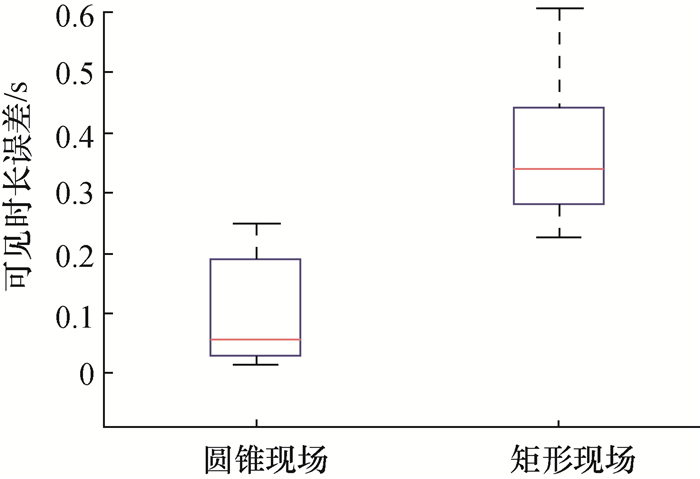图 6 计算结果与STK结果偏差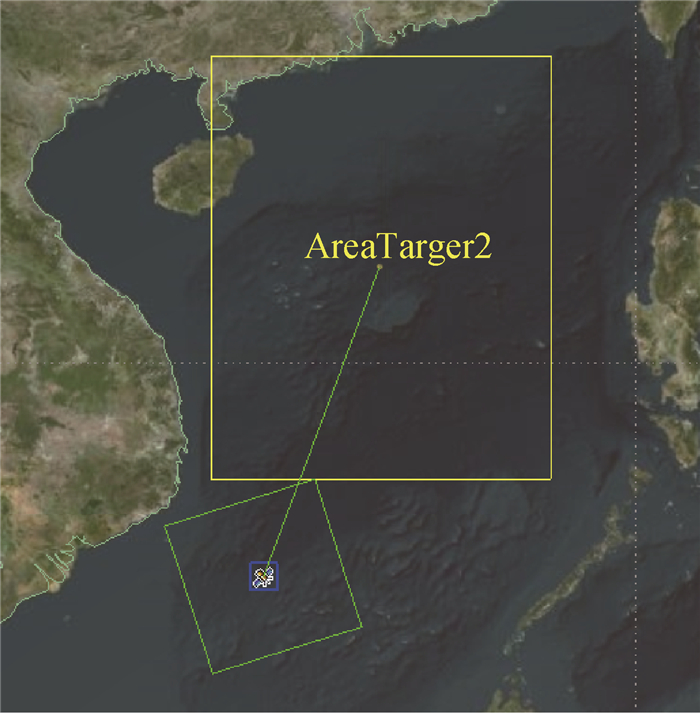图 7 矩形视场与区域目标位置关系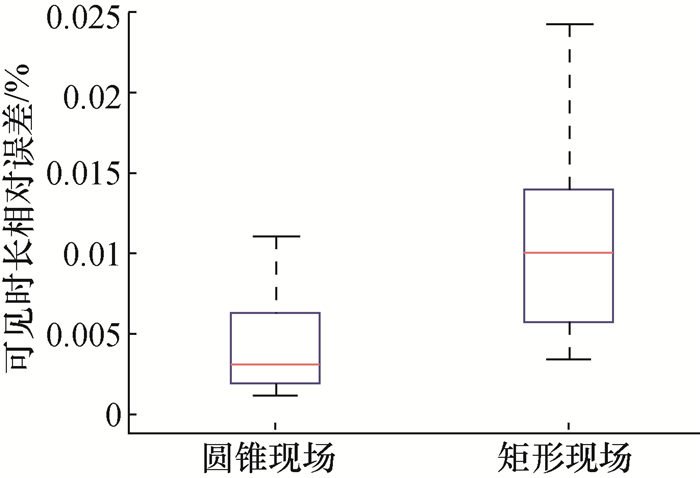图 8 修正计算结果与STK结果偏差